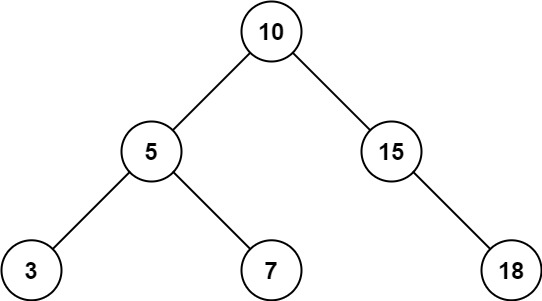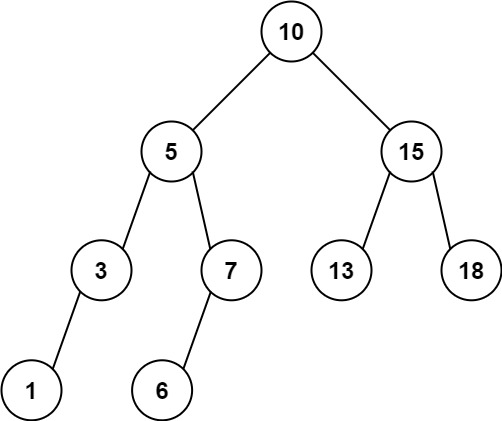Version: v1.6.5 Latest LatestGo to latest
Published: Mar 23, 2021 License: MIT

##README ¶

### 938. Range Sum of BST (Easy)

Given the `root` node of a binary search tree, return the sum of values of all nodes with a value in the range `[low, high]`.

Example 1:```Input: root = [10,5,15,3,7,null,18], low = 7, high = 15
Output: 32
```

Example 2:```Input: root = [10,5,15,3,7,13,18,1,null,6], low = 6, high = 10
Output: 23
```

Constraints:

• The number of nodes in the tree is in the range `[1, 2 * 104]`.
• `1 <= Node.val <= 105`
• `1 <= low <= high <= 105`
• All `Node.val` are unique.

[Tree] [Depth-first Search] [Recursion]

##Documentation ¶

### Constants ¶

This section is empty.

### Variables ¶

This section is empty.

### Functions ¶

This section is empty.

### Types ¶

#### type TreeNode ¶

`type TreeNode = kit.TreeNode`

TreeNode - Definition for a binary tree node.